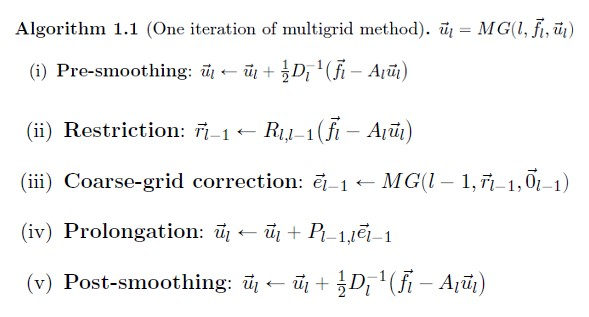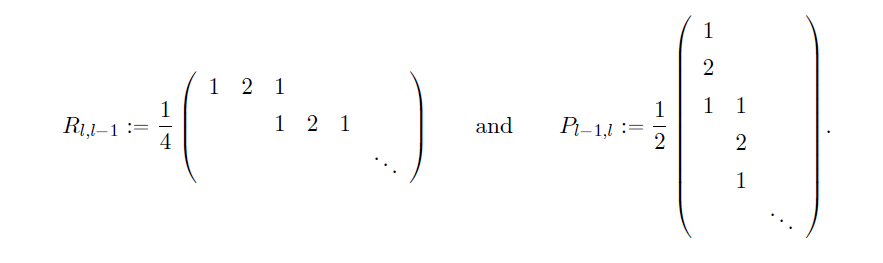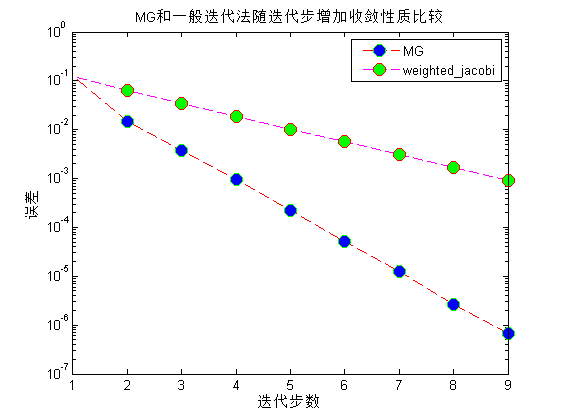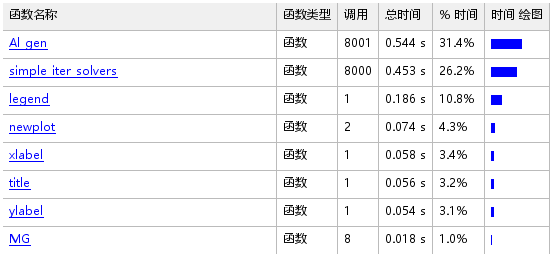# 多重网格方法

## 多重网格方法

### 算法过程

• 预平滑
选定一个初值，先采用一般方法，比如说权雅克比方法迭代一两次，求出一个近似解 u l u_l
• 粗网格校正
1、计算剩余量 r l = f l − A l u l r_l = f_l - A_lu_l
2、限制剩余量 r l − 1 = R l , l − 1 r l r_{l-1}=R_{l,l-1}r_l ，其中的 R R 为限制算子，它实质上是一个插值算子。
3、在粗网格上，求下列问题精确解：
A l − 1 ∗ e l − 1 = r l − 1 A_{l-1}* e_{l-1}= r_{l-1}
其中， A l − 1 A_{l-1} 是在粗网格上离散后得到的矩阵（如果是有限元方法，就是刚度矩阵）。如果在这个粗网格上精确解不好求，继续放粗，递归调用整个过程。
4、将 e l − 1 e_{l-1} 延拓到粗网格上，以求解 e l e_l e l = P l − 1 , l e l − 1 e_l = P_{l-1,l}e_{l-1} ，其中 P P 为延拓算子，其实它是一个插值算子。
5、计算粗网格上的近似解，仍然用 u l ul 表示：
u l = u l + e l u_l = u_l + e_l
• 后平滑
以算得的 u l u_l 为初值，采用一般迭代法再迭代个一两次，求解近似解。### 数值实验与结果clc
clear
l = 5;
fl = fl_gen(l);
N = 2^(l+1) - 1;
u0 = ones(N,1);
u_true = Al_gen(l)\fl;
m = 8;%算法迭代次数
ul = u0;
error_MG = norm((u_true - ul),2)/N;
for i=1:m
ul = MG(l,fl,ul);
error_MG(end+1) = norm((u_true - ul),2)/N;
end;

mm = 1000;
method = 'weighted_jacobi';%Richardson weighted_jacobi Gauss_Seidel SOR
w = 1/2;
ul = u0;
error_si_sol = norm((u_true - ul),2)/N;
for i = 1:m
for j = 1:mm
options = struct('A',Al_gen(l),'u_old',ul,'f',fl,'method',(method),'w',w);
ul = simple_iter_solvers(options);
end
error_si_sol(end+1) = norm((u_true - ul),2)/N;
end

p1 = semilogy(error_MG);
hold on;
p2 = semilogy(error_si_sol);
legend('MG','weighted\_jacobi')
set(p1,'MarkerFaceColor',[0 0 1],'MarkerEdgeColor',[0 1 0],...
'MarkerSize',10,...
'Marker','o',...
'LineStyle','--',...
'Color',[1 0 0],...
'DisplayName','MG');
set(p2,'MarkerFaceColor',[0 1 0],'MarkerEdgeColor',[1 0 0],...
'MarkerSize',10,...
'Marker','o',...
'LineStyle','--',...
'Color',[1 0 1]);

xlabel('迭代步数');
ylabel('误差');
title('MG和一般迭代法随迭代步增加收敛性质比较');

function ul = MG(l,fl,ul)
Al = Al_gen(l);
%rl = fl-Al*ul;
%if norm(rl,2)/norm(fl,2) < 10e-6 || l<1
if l == 1
%  ul = zeros(2^(l+1)-1,1);
% fl = fl_gen(1);
ul = Al\fl;
return;
end
%% 迭代方法的选择
method = 'weighted_jacobi';%可以改成其他方法Gauss_Seidel weighted_jacobi

%% 预平滑
%for i = 1:4
ul0 = ul;
options = struct('A',Al,'u_old',ul,'f',fl,'method',method,'w',1/2);
ul = simple_iter_solvers(options);
%end
%% 限制
[Pl,Rl] = PR_gen(l);
rl_1 = Rl*(fl-Al*ul0);
%rl_1 = Rl*(fl-Al*ul);
%% 粗网格校正
el_1 = MG(l-1,rl_1,zeros(2^l-1,1));
%% 延拓
ul = ul + Pl*el_1;
%% 后平滑
%for i = 1:4
options = struct('A',Al,'u_old',ul,'f',fl,'method',method,'w',1/2);
ul = simple_iter_solvers(options);
%end
end

function u_new = simple_iter_solvers(options)
%帮助信息：
%输入参数为options = struct('A',A,'u_old',u_old,'f',f,'method','method','w',w);
if nargin < 1, help(mfilename),
printf('输入参数错误。')
end
%options = varargin;
A = options.A;
u_old = options.u_old;
f = options.f;
method = options.method;
D = diag(diag(A));
L = tril(A,-1);
U = triu(A,1);

if strcmp(method,'Richardson')==1
w = options.w;
u_new = u_old + w*(f-A*u_old);
end
if strcmp(method,'weighted_jacobi')==1
w = options.w;
%   u_new = u_old + w*D^-1*(f-A*u_old);
u_new = u_old + w*(D\(f-A*u_old));
%    u_new = u_new/4;
end
if strcmp(method,'Gauss_Seidel')==1
u_new = u_old + (D+L)\(f-A*u_old);
end
if strcmp(method,'SOR')==1
w = options.w;
u_new = (D+w*L)\(w*f - (w*U+(w-1)*D)*u_old);
end

end

function [P,R] = PR_gen(l)
% 我们用PR_gen来生成限制算子R和磨光算子P，输入参数l表示较细网格划分了l次
N = 2^(l+1) - 1;
%h = 1/2^(l+1);
N_ = 2^l- 1;
%h_ = 1/2^(l);
p = 1/2*[1;2;1;zeros(N-3,1)];
P = zeros(N,N_);
for i = 0:N_-1
P(:,i+1) = P(:,i+1) + circshift(p,2*i);
end
R = 1/2*P';
end

function fl = fl_gen(l)
NN = 2^(l+1);
h = 1/NN;
x = h:h:1-h;
fl = fun(x);
end
function fx = fun(x)
fx = sin(2*pi*x);
fx = fx';
%fx = ones(length(x),1);
%fx = zeros(length(x),1);
end

function A = Al_gen(l)
%% Al生成器
N = 2^(l+1) - 1;
h = 1/2^(l+1);
A = (1/h^2)*(diag(repmat(-1,N-1,1),-1)+diag(repmat(2,N,1))+diag(repmat(-1,N-1,1),1));
end### 其它一些东西

1、之前程序问题了，花了好长时间查错。后来发现一直用w*A\bw*(A^-1*b)，这是不对的。因为w*A\b等价于(w*A)\b，求逆符号\和乘号*是同级的，是按从左往右算，一个一个括号的问题，耗费了我大量时间。

2、在求Restriction步骤时，我发现 R l , l − 1 ( f l − A l u l ) R_{l,l-1}(f_l-A_lu_l) 中的 u l u_l 使用预平滑之前的值，即初值，结果会更精确，我并不知道为什么。

3、matlab中semilogy函数，只不过是在刻度显示上，关于指数均匀增长，而y值是没有改变的。semilogy控制的只是坐标轴的剖分，按照指数等刻度均匀划分，并没有实际对y值取log。

08-11
03-2011-011107
05-25380
02-031293
10-15183
02-03652
03-04
01-194万+